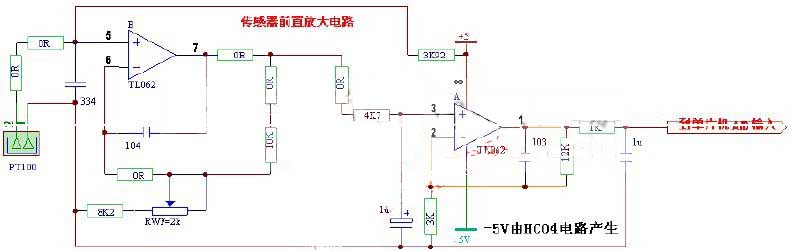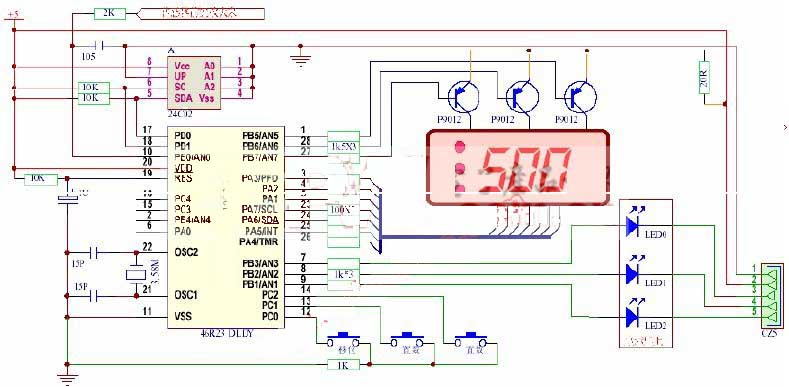﻿ Circuit Design and Formula Calculating Method for Conventional Temperature Measurement Range of PT100 Platinum Resistance Sensor_

China sensor manufacturers

# Circuit Design and Formula Calculating Method for Conventional Temperature Measurement Range of PT100 Platinum Resistance Sensor

Pt100 temperature measurement circuit (classic temperature range): The temperature sensor PT100 can work in the range of -200 degrees to 650 degrees. The whole circuit is divided into two parts, one is the sensor preamplifier circuit, the other is the part of the single chip AD conversion and the display control software nonlinear correction.Pt100 sensor preamplifier circuitThe Circuit Principle of PT100 Single Chip Microcomputer

PT100 calculation formula:
Thermal resistance is the most commonly used temperature detector in the low temperature zone. Its main features are high measurement accuracy and stable performance. The following is my own calculation formula in the microcontroller program:
A: The formula given in the relevant materials:
1. Temperature characteristics of platinum thermal resistance. In the range of 0~850 °C
Rt=R0(1+At+Bt2)
In the range of -200~0 °C
Rt=R0[1+At+Bt2+C(t-100)t3]
The coefficients of A, B, and C in the formula are:
A=3.90802×10^-3°C-1
B=-5.802×10^-7°C-2
C=-4.27350×10^-12°C-4
2. Temperature characteristics of copper thermal resistance: in the range of -50~150 °C
Rt=R0[1+At+Bt2+Ct3]
A=4.28899×10^-3°C^-1
B=-2.133×10^-7°C^-2
C=1.233×10^-9°C^-3

Second, the calculation formula I used in the program:
2. Temperature measurement technology (PT100):
When T 0
RT=Rt
When T > 420
RT= Rt+ Rt2*2.15805393*10-6
When 0T 420
RT= Rt*[1+(R420-Rt)*3.301723797*10-7]+ Rt2*2.15805393*10-6
Correlation coefficient and description:
RT is a linear value corresponding to temperature, and the result is equivalent to the display temperature value.
Rt is the actually measured impedance value, and its value is the value that has been subtracted by 100 (the reference value of the bridge differential).